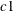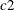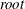The COPULA Procedure

DEFINE Statement

• DEFINE name copula-type < ( parameter-value-options ... ) > ;

The DEFINE statement specifies the relevant information of the copula used for the simulation.

name

specifies the name of the copula definition, which can be used later in the SIMULATE statement.

copula-type

specifies one of the following types of the copula:

NORMAL

specifies the normal copula.

T

specifies the t copula.

CLAYTON

specifies the Clayton copula.

GUMBEL

specifies the Gumbel copula.

FRANK

specifies the Frank copula.

HACCLAYTON

specifies the hierarchical Clayton copula.

HACGUMBEL

specifies the hierarchical Gumbel copula.

HACFRANK

specifies the hierarchical Frank copula.

These copula models are also described in the section Details: COPULA Procedure.

parameter-value-options

specify the input parameters used to simulate the specified copula. These options must be appropriate for the type of copula specified. The following options are valid:

CORR=SAS-data-set

specifies the data set that contains the correlation matrix to use for elliptical copulas. If the correlation matrix is valid but not submitted in order, then you must provide the variable names in the first column of the matrix and these names must match the variable names in the VAR statement. See Output 10.2.1 for an example of a correlation matrix input in this form. If the correlation matrix is submitted in order, the first column of variable names is not required. This option can be used for the normal and t copulas.

KENDALL=SAS-data-set

specifies the data set that contains the correlation matrix defined in Kendall’s tau. If the correlation matrix is valid but not submitted in order, then you must provide the variable names in the first column of the matrix and these names must match the variable names in the VAR statement. If the correlation matrix is submitted in order, the first column of variable names is not required. This option can be used for the normal and t copulas.

SPEARMAN=SAS-data-set

specifies the data set that contains the correlation matrix defined in Spearman’s rho. If the correlation matrix is valid but not submitted in order, then you must provide the variable names in the first column of the matrix and these names must match the variable names in the VAR statement. If the correlation matrix is submitted in order, the first column of variable names is not required. This option can be used for the normal copula.

DF=value

specifies the degrees of freedom. This option can be used for the t copula.

THETA=value

specifies the parameter value for the Archimedean copulas.

HIERARCHY=(name=(HAC-specification)(THETA=value))

specifies the hierarchy for hierarchical Archimedean copulas. The argument usually consists of multiple specification lines, with each line specifying one copula in the hierarchy. name can be user-defined symbols, with the exception of the copula at the top of the hierarchy, which must be named ROOT. The HAC-specification is a list of symbols that can be either defined copula names or variable names from the VAR statement, depending on whether the element of the copula is a variable or an inner copula in the hierarchy. For example, you can use the following code to define a hierarchical Archimedean copula, with the hierarchy shown in Figure 10.5:

```   var u1-u4;
define cop hacclayton hierarchy=(
root = (c1 c2)(theta=1)
c1 = (u1 u2)(theta=3)
c2 = (u3 u4)(theta=5));
```

Note that as long as the specification is valid, the order of the specification lines does not matter. In the previous example, you could first listand, and then define.

The DEFINE statement is used with the SIMULATE statement.The FIT statement can also be used with the SIMULATE statement. The results of the FIT statement can be the input of the SIMULATE statement. Therefore, the SIMULATE statement can follow the FIT statement.If there is no FIT statement, then the DEFINE statement must precede SIMULATE statement. However, the FIT and DEFINE statements cannot both be used in the same procedure.